# MHD Mixed Bioconvection in a Square Porous Cavity Filled by Gyrotactic Microorganisms

MHD Mixed Bioconvection in a Square Porous Cavity Filled by Gyrotactic Microorganisms

Mohamed A. Mansour Ahmed M. Rashad Bandaru Mallikarjuna Ahmed Kadhim Hussein Mohamed Aichouni Lioua Kolsi

Department of Mathematics, Assuit University, Faculty of Science, Assuit 71515, Egypt

Department of Mathematics, Aswan University, Faculty of Science, Aswan 81528, Egypt

Department of Mathematics, BMS College of Engineering, Bangalore 560019, India

College of Engineering, Mechanical Engineering Department, Babylon University, Babylon City, Hilla 51002, Iraq

College of Engineering, Industrial Engineering Department, Hail University, Hail City 2240, Saudi Arabia

College of Engineering, Mechanical Engineering Department, Hail University, Hail City 2240, Saudi Arabia

Laboratoir de Métrologie et des Systèmes Énergétiques, École Nationale d’Ingénieurs, University of Monastir, Monastir 5000, Tunisia

Corresponding Author Email:
lioua_enim@yahoo.fr
Page:
433-445
|
DOI:
https://doi.org/10.18280/ijht.370209
30 December 2017
|
Accepted:
29 April 2019
|
Published:
30 June 2019
| Citation

OPEN ACCESS

Abstract:

A numerical investigation of magneto-hydrodynamic mixed convection in a square lid-driven cavity filled with gyrotactic micro-organisms is examined. Different magnetic field inclinations are considered and the left wall of the lid-driven cavity is moving up with a constant speed in the vertical direction. The left and right vertical walls of the cavity are assumed to be adiabatic. The top horizontal wall of the cavity is assumed to be kept at features of the solution. Lower temperature, while the bottom horizontal wall is kept in a higher temperature. The developed equations of the mathematical model are put in their dimensionless forms and then solved numerically subject to appropriate boundary conditions by the control finite volume method. Comparisons with previously published works are presented and found to be in excellent agreement. The problem was studied by varying the Richardson number (Ri=0.01-2.0), Rayleigh number (Ra=102 -105), magnetic field inclination angle (Φ=0o-350o), Hartman number (Ha=0–50), and bio-convection Rayleigh number (Rb=10-40). A parametric study is performed and a set of graphical results is presented and discussed to demonstrate interesting.

Keywords:

MHD, mixed convection, square enclosure, gyrotactic microorganisms

1. Introduction

Mixed convection flow is accomplished in diverse transport processes and thermal engineering applications including lubrication technologies, cooling of electronic equipment, high-performance building isolation, multi-shield structures utilized for nuclear reactors, food processing, ovens, solar power collectors, glass manufacture, drying technologies, chemical processing devices and others [1-2]. A concise review of literature exhibits that the analysis of mixed convection heat transfer in cavities has attracted noticeable attention in the past few decades [3-9]. Also, there has been boosted interest in examining buoyancy motivated flow by heat transfer from various geometries saturated porous media. This concern stems from different engineering and geophysical applications like as thermal insulation, geothermal tanks, improved oil recovery, drying of porous solids, cooling of nuclear reactors, packed bed catalytic reactors and underground energy transport. References of overall literature studies concerning the object of porous media can be had in most recent books by [10-13].

Bioconvection is an interest biological swimming mechanism resulting from complex interactions between phenomena at several physical scales. The procedure is driven by the trend of self-propelled micro-organisms that are intensive than the suspension fluid [14-15]. The swimming of each individual micro-organism is a mesoscale physical phenomenon. Micro-organisms characteristically swim in the upwards trend, leading to an uneasy, upper-heavy density stratification, which under specific stipulations may result in hydrodynamic instability. Bioconvection has been shown to offer the potential to improve mass transport and induce combining, especially in microvolumes, and enhance the stability of fluids. Kuznetsov  and several other authors have examined different kinds of bioconvection flows. He performed a continuum model for thermos-bioconvection of oxytactic bacteria in a porous media to study the impacts of microorganisms’ up swimming and heating from below on the stability of bioconvection in a horizontal layer. Bég et al.  considered the problem of natural convection magneto-micropolar biopolymer flow over a horizontal circular cylinder. Alloui et al.  analyzed the influence of heating/cooling from bottom on the stability of a suspension of motile gravitactic microorganisms in a shallow fluid layer. Alloui et al.  have also examined the impact of heating/cooling from below on the evolution of gravitactic bioconvection in a square cavity. Kuznetsov  established a theory for bio-thermal convection in a suspension that contains two species of microorganisms demonstrating various taxes, gyrotactic and oxytactic microorganisms. Kuznetsov et al.  presented a theoretical investigation of a falling bioconvection plume in a chamber filled with a fluid saturated porous medium. Kuznetsov  discussed the influence of below heating on the stability of a suspension of motile gyrotactic microorganisms in a fluid layer of finite depth. He observed that a suspension of gyrotactic microorganisms in a horizontal fluid layer heated from below is less stable than the same suspension under isothermal conditions. Other investigation on thermos-bioconvection can be found in refs. [23-26].

The main goal of this investigation is to examine the inclined magneto-hydrodynamic mixed convection in a porous square lid-driven cavity filled with a gyrotactic micro-organisms employing finite volume method. The outcomes are exhibited with flow configurations, isotherms, concentration contours as well as the average Nusselt and Sherwood numbers for different cases. The outcomes of this study are well validated and have favorable comparisons with previously published data. The applications of such type of these problems can be established in highly active fuel cells where thermophilic microorganisms, like as Bacillus licheniformis and Bacillus thermoglucosidasius are applied. Also, it is pertinent in applications like motile thermophilic microorganisms that live in warm springs.

2. Geometry and Mathematical Model

Consider a steady two-dimensional mixed convection flow inside a porous square lid-driven cavity of length H filled with gyrotactic microorganism, as shown in Figure 1. The coordinates x and y are chosen such that x measures the distance along the bottom horizontal wall, while y measures the distance along the left vertical wall, respectively. The left and right walls are assumed to be adiabatic. The left wall is moving up with constant speed in vertical direction V0. In order to induce the buoyancy effect, the top side horizontal wall of the cavity is kept at a lower constant temperature Tc, while the bottom horizontal wall is kept in a higher temperature Th, where Th>Tc. It is also assumed that the induced magnetic field produced by the motion of an electrically conducting nanofluid is negligible compared to the applied magnetic field. The gravitational acceleration acts downward. The direction uniform magnetic field with a constant magnitude B0 is applied in the angle F with the horizontal direction. It is further assumed that both the fluid phase and nanoparticles are in thermal equilibrium. Except for the density the properties of nanoparticles and fluid are taken to be constant. Moreover, many bacterial species swim up the oxygen gradients, and this behavior is called oxytaxis. Oxytactic bacteria require oxygen for their metabolism and swim up the oxygen gradients. They require a minimum oxygen concentration Cmin to be active. The nanoparticle suspension is assumed to be stable. It is also assumed, as in Kuznetsov , that the presence of nanoparticles has no effect on the direction of microorganisms swimming or on their swimming velocity. Bio-convection-induced flow is expected to occur only in a dilute suspension of nanoparticles. If this is not the case, then a large concentration of nanoparticles would result in a large suspension viscosity, which would suppress bio-convection. The thermo-physical properties of the fluid are assumed constant except for the density variation, which is determined based on the Boussinesq approximation. Under the above assumptions and following Hillesdon and Pedley , the governing equations embodying the conservation of the total mass, momentum, thermal energy, concentration, and micro-organisms can be written as; the flow, heat and mass transfer, see Kuznetsov .

$\frac{\partial u}{\partial x}+\frac{\partial v}{\partial y}=0$  (1)

$u\frac{\partial u}{\partial x}+v\frac{\partial u}{\partial y}=-\frac{1}{{{\rho }_{f}}}\frac{\partial p}{\partial x}+\upsilon \left( \frac{{{\partial }^{2}}u}{\partial {{x}^{2}}}+\frac{{{\partial }^{2}}u}{\partial {{y}^{2}}} \right)-\frac{\upsilon }{K}u+\frac{\sigma \,B_{0}^{2}}{{{\rho }_{f}}}\left( v\,\sin \Phi \,\cos \Phi -u{{\sin }^{2}}\Phi \right)$ (2)

$u\frac{\partial v}{\partial x}+v\frac{\partial v}{\partial y}=-\frac{1}{{{\rho }_{f}}_{{}}}\frac{\partial p}{\partial y}+\upsilon \left( \frac{{{\partial }^{2}}v}{\partial {{x}^{2}}}+\frac{{{\partial }^{2}}v}{\partial {{y}^{2}}} \right)\,-\frac{\upsilon }{K}v+\frac{\sigma \,B_{0}^{2}}{{{\rho }_{f}}}\left( u\sin \Phi \cos \Phi -v{{\cos }^{2}}\Phi \right)-\frac{1}{{{\rho }_{f}}}[\gamma \Delta \,\rho n-{{\rho }_{f}}\beta (T-{{T}_{c}})]g$ (3)

$u\frac{\partial T}{\partial x}+v\frac{\partial T}{\partial y}=\alpha \left( \frac{{{\partial }^{2}}T}{\partial {{x}^{2}}}+\frac{{{\partial }^{2}}T}{\partial {{y}^{2}}} \right)$ (4)

$u\frac{\partial C}{\partial x}+v\frac{\partial C}{\partial y}={{D}_{c}}\left( \frac{{{\partial }^{2}}C}{\partial {{x}^{2}}}+\frac{{{\partial }^{2}}C}{\partial {{y}^{2}}} \right)-\delta \,n$   (5)

$\frac{\partial }{\partial x}\left[ un+\tilde{u}n-{{D}_{n}}\frac{\partial n}{\partial x} \right]+\frac{\partial }{\partial y}\left[ vn+\tilde{v}n-{{D}_{n}}\frac{\partial n}{\partial y} \right]=0\,$ (6)

The boundary conditions are:

On the left wall: $x=0, v=V_{0}, u=0, \frac{\partial T}{\partial x}=0, \frac{\partial C}{\partial x}=0, \frac{\partial n}{\partial x}=0$

On the right wall: $x=1, v=0, u=0, \frac{\partial T}{\partial x}=0, C=C_{0} \frac{n b W_{c}}{\Delta C} \frac{\partial C}{\partial x}-D_{n} \frac{\partial n}{\partial x}=0$

On the bottom wall: $y=0, v=0, u=0, T=T_{h}, C=C_{0}, n=n_{0}$

On the top wall: $y=0, v=0, u=0, T=T_{c}, C=C_{\min }, n=n_{0}$  (7)

In the above equations $\tilde{u}=(\frac{b\,{{W}_{C}}}{\Delta \,C})\,\frac{\partial \,C}{\partial \,x}$ and $\tilde{v}=(\frac{b\,{{W}_{C}}}{\Delta \,C})\frac{\partial \,C}{\partial \,y}$; where x and y are the Cartesian Co-ordinates measured in the horizontal and vertical directions, respectively; u and v are the velocity components along the x and y-axes; T, C are the temperature and concentration; K is the permeability of the porous medium; $\mu$ is the dynamic viscosity of the suspension  (the suspension includes fluid plus microorganisms); $\gamma$ is the average volume of a microorganisms; $\Delta \rho \,={{\rho }_{cell}}\,-{{\rho }_{f}}$ is the density difference between cells and fluid; ${{\rho }_{f}}$ is the density of the fluid; n is the number density of motile microorganisms; β is the volume expansion coefficient of water at constant pressure; g is the gravity; α is the effective thermal diffusivity of the porous medium; Cp is the specific heat at constant pressure, DC is the diffusivity of oxygen; Dn is the diffusivity of the microorganisms; $-\delta \,n$ describes the consumption of the microorganisms; Cmin is the minimum oxygen concentration that microorganisms need in order to be active; b is the chemotaxis constant, WC is the maximum cell swimming speed (the product bWC is assumed to be constant ).

Introducing the following dimensionless set:

$X=\frac{x}{H}, Y=\frac{y}{H}, U=\frac{u}{V_{0}}, V=\frac{v}{V_{0}}, P=\frac{p}{\rho V_{0}^{2}}, \theta=\frac{(T-T c)}{\Delta T}$

$N=\frac{n}{n_{0}}, \phi=\frac{C-C_{\min }}{\Delta C}, \Delta T=\left(T_{h}-T_{c}\right), \Delta C=\left(C_{0}-C_{\min }\right), \chi=\frac{D_{c}}{D_{n}}$  (8)

## 1.png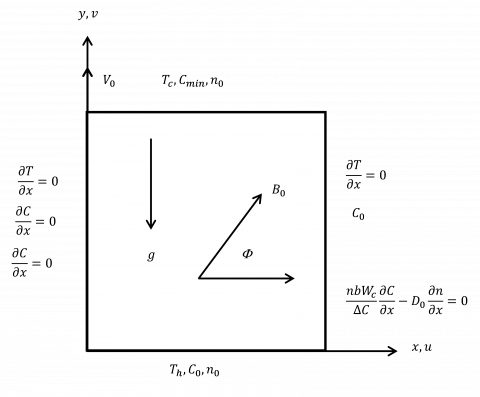Figure 1. Schematic diagram of the problem under consideration

into Eqns. (2)-(6) yields the following dimensionless equations:

$U\frac{\partial U}{\partial X}+V\frac{\partial U}{\partial Y}=-\frac{\partial P}{\partial X}+\frac{1}{\operatorname{Re}}\left( \frac{{{\partial }^{2}}U}{\partial {{X}^{2}}}+\frac{{{\partial }^{2}}U}{\partial {{Y}^{2}}} \right)-\frac{U}{Da\operatorname{Re}}+\frac{H{{a}^{2}}}{\operatorname{Re}}\left( V\sin \Phi \cos \Phi -U{{\sin }^{2}}\Phi \right)$   (9)

$U\frac{\partial V}{\partial X}+V\frac{\partial V}{\partial Y}\,=-\frac{\partial P}{\partial Y}+\frac{1}{\operatorname{Re}}\left( \frac{{{\partial }^{2}}V}{\partial {{X}^{2}}}+\frac{{{\partial }^{2}}V}{\partial {{Y}^{2}}} \right)-\frac{V}{Da\operatorname{Re}}+\frac{H{{a}^{2}}}{\operatorname{Re}}\left( U\sin \Phi \cos \Phi -V{{\cos }^{2}}\Phi \right)\,\,+Ri\,\left( \theta -RbN \right)$   (10)

$U\frac{\partial \theta }{\partial X}+V\frac{\partial \theta }{\partial Y}=\frac{1}{\Pr \operatorname{Re}}\,\,{{\nabla }^{2}}\theta$  (11)

$U\frac{\partial \phi }{\partial X}+V\frac{\partial \phi }{\partial Y}=\frac{1}{Sc\operatorname{Re}}{{\nabla }^{2}}\phi -\frac{{{\sigma }_{1}}}{Sc\operatorname{Re}}N$  (12)

$\frac{1}{\operatorname{Re}}{{\nabla }^{2}}N=Sc\chi \,\left( U\frac{\partial N}{\partial X}+V\frac{\partial N}{\partial Y} \right)+\frac{Pe}{\operatorname{Re}}\left( N{{\nabla }^{2}}\phi +\frac{\partial N}{\partial X}\frac{\partial \phi }{\partial X}+\frac{\partial N}{\partial Y}\frac{\partial \phi }{\partial Y} \right)$     (13)

where $\,Ra=\frac{gK\beta \Delta T{{H}^{3}}}{{{\upsilon }^{2}}}\,\,,Rb=\frac{\gamma \Delta \rho \,{{n}_{0}}}{{{\rho }_{f}}\beta \Delta T},Pe=\frac{b{{W}_{C}}}{{{D}_{n}}},\Pr =\frac{\upsilon }{\alpha },Sc=\frac{\upsilon }{{{D}_{c}}}$, $Ha={{B}_{0}}H\sqrt{\sigma /\mu }$, $Da=\frac{K}{{{H}^{2}}}$, $\operatorname{Re}=\frac{{{V}_{0}}H}{\upsilon}$, ${{\sigma}_{1}}=\frac{\delta \,{{n}_{0}}\,{{H}^{2}}}{{{D}_{c}}\,\Delta C}$, $Ri=\frac{Gr}{{{\operatorname{Re}}^{2}}}$ are the Rayleigh number, bioconvection Rayleigh number, bioconvection Peclet number, Prandtl number, Schmidt number, Hartmann number, Darcy number, Reynolds number, ratio of the rate of oxygen consumption to the rate of oxygen diffusion, and Richardson number, respectively.

The dimensionless boundary conditions are

On the left wall: $X=0, V=1, U=0, \frac{\partial \theta}{\partial X}=0, \frac{\partial \phi}{\partial X}=0, \frac{\partial N}{\partial X}=0$

On the right wall: $X=1, U=0, V=0, \frac{\partial \theta}{\partial X}=0, \phi=1, P e N \frac{\partial \phi}{\partial X}-\frac{\partial N}{\partial X}=0$

On the bottom wall: $Y=0, V=0, U=0, \theta=1, \phi=1, N=1$

On the top wall: $Y=0, V=0, U=0, \theta=0, \phi=0, N=1$  (14)

The local Nusselt and Sherwood numbers at the heated wall are defined

$N{{u}_{Y}}=-{{\left( \frac{\partial \theta }{\partial Y} \right)}_{Y=0}},S{{h}_{Y}}=-{{\left( \frac{\partial \phi }{\partial Y} \right)}_{Y=0}}$   (15)

The average Nusselt and Sherwood numbers are, also, defined as follows;

$N{{u}_{m}}=\int\limits_{0}^{1}{N{{u}_{Y}}dX},S{{h}_{m}}=N{{u}_{m}}=\int\limits_{0}^{1}{S{{h}_{Y}}dX}$.  (16)

3. Numerical Method and Validation

Equations (9)-(13) with the boundary conditions (14) have been solved numerically using the collocated finite volume method. The first upwind and central difference approaches have been used to approximate the convective and diffusive terms, respectively. The resulting discretized equations have been solved iteratively, through alternate direction implicit ADI, using the SIMPLE algorithm . The velocity correction has been made using the Rhie and Chow interpolation. For convergence, under-relaxation technique has been employed. To check the convergence, the mass residue of each control volume has been calculated and the maximum value has been used to check the convergence. The convergence criterion was set as 10-5. In order to verify the accuracy of the present method, the obtained results in special cases are compared with the results obtained by Iwatsu et al.  and Khanafer & Chamkha  in terms of the mean Nusselt number at the top wall, for different values of Re. As we can see form Table 1, the results are found in a good agreement with these results. These favorable comparisons lend confidence in the numerical results to be reported subsequently.

Figure 2 displays a comparison between the temperature contour presented in this work with those of Iwatsu et al.  and Khanafer & Chamkha  and. The result shows a very good agreement between this work and the previously published work.

Table 1. Comparisons of the mean Nusselt number at the top wall, for different values of Re at Pr=0.71, Ra=102

 Re Iwatsu et al.  Khanafer & Chamkha  Present study 100 1.94 2.01 1.93 400 3.84 3.91 3.91 1000 6.33 6.33 6.31

## 2.png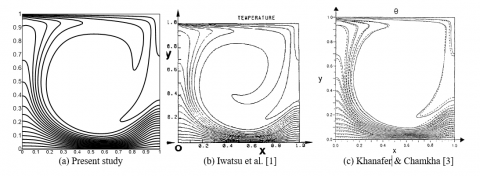Figure 2. Comparison of the present study (a) with results of Iwatsu et al.  (b) and Khanafer & Chamkha  (c) for $R e=1000, P r=0.71, R a=10^{2}$ and $H a=R b=0$

4. Results and Discussions

4.1 Effect of the Richardson number on streamlines, isotherms, concentrations and isolines of micro- rotation

The streamlines (first column) , isotherms (second column) , concentrations (third column) and isolines of micro- rotation (fourth column) in the square cavity filled with a gyrotactic micro-organisms are shown in Figure 3 when the Richardson number varies from 0.01 to 2 and [Φ=0o , Ra=104, Rb=1, s1=1, Sc=1, Pr=6.2, Da=10-3 and χ=1].

4.1.1 The forced convection effect (Ri=0.01 and 0.5)

Figure 3a and Figure 3b illustrate the streamlines, isotherms, iso-concentrations and isolines of micro-rotation when the forced convection effect is dominated [i.e., Ri=0.01 and 0.5] as shown in the first and second rows of these figures respectively.

In this case, the shear force due to the moving lid-driven left sidewall has a greater effect than the buoyancy force which is generated due to the temperature difference between the top and bottom walls. It can be clearly seen that when the left sidewall moves towards the upward direction, the large rotating single vortices begin to move upward and occupy the most size of the cavity. This is because the convection currents begin from the hot bottom wall and move towards the top cold wall due to both buoyancy and shear forces. Also, it can be seen that the absolute values of the stream function begin to decrease as the Richardson number increases. Isotherms are clustered near of the hot bottom wall of the cavity due to the severe temperature gradients in the vertical direction which gives an indication that the convection heat transfer becomes more significant compared to the conduction one. Thus for very low Richardson numbers is (i.e., Reynolds number is high), isotherm contours have a clear disturbance so the convection heat transfer is dominant. With respect to the iso-concentration contours, it can be seen that they are clustered adjacent the hot bottom and cold top walls of the cavity. Similar behavior to isotherm contours can be seen where the concentration contours refer that the heat diffuses inside the porous cavity by the concentration gradients between the bottom and the top walls. Moreover, it can be seen from the results that the concentrations adjacent the hot bottom wall are greater than their values at the cold top one and satisfies indirectly the validity of the problem boundary conditions. With respect to the isolines of microrotation, it can be seen from the results of Figure 3a and Figure 3b that as the Richardson number increases from [Ri=0.01 to 0.5], the strength of the microrotation decreases in the core of the cavity. A reverse behavior can be noticed adjacent the hot bottom wall.

4.1.2 The mixed convection effect (Ri=1.0)

Figure 3c shows the streamlines, isotherms, iso-concentrations and isolines of micro-rotation for balanced forced and natural convections (i.e., Ri=1). In this case, the shear force is equivalent to the buoyancy force. It can be seen from results that the behavior of the flow field is approximately similar to that observed at [Ri=0.5]. The only difference is that the rotating vortices begin to further extended towards the bottom wall and their eye are elongated compared with the corresponding vortices at [Ri=0.5]. Regarding isotherms and iso-concentrations, a similar pattern to that found in [Ri=0.5] occurs, except that both of them are more distributed inside the cavity especially beside the top horizontal wall. The same behavior can be noticed also with respect to the isolines of microrotation. The only difference which is that the strength of microrotation are increased at [Ri=1] compared to their corresponding values at [Ri=0.5].

4.1.3 The natural convection effect (Ri=2.0)

Figure 3d presents the streamlines, isotherms, iso-concentrations and isolines of micro-rotation when the natural convection effect is greater than the forced convection effect (i.e., Ri=2.0). In this case, the buoyancy force has a strong effect compared with the shear force. Also, it can be noticed that the effect of the left side lid-driven wall becomes weak and the buoyancy force tries to push the rotating vortices towards the bottom wall. Moreover, the circulation intensity increases slightly due to the decrease in the shear force and the increase in the buoyancy force when the Richardson number increases from 1.0 to 2.0. The increase in Richardson number decreases the effect of the left side lid-driven wall and causes to increase the circulation intensity. The maximum absolute value of the circulation intensity for this case is about (0.022). For isotherms and iso-concentrations, it is observed that they are distributed irregularly inside the cavity as curved lines; in this case the convection heat transfer is more dominant. This result is due to the high temperature and concentration gradients for such the Richardson numbers which causes the enhancement of the rates of heat and mass transfer. In addition, when the Richardson number is high, the thermal and solutal buoyancy forces effect due to the natural convection increases and the transferred heat and mass increase also which leads to increase the rate of heat and mass transfer inside the cavity. It can be seen from the results of Figure 3d that for [Ri=2], the strength of the microrotation decreases again in the core of the cavity, while it increases adjacent the hot bottom wall compared to [Ri=1] case.

4.2 Effect of the Richardson number on the local Nusselt number

Figure 4 shows the profiles of the local Nusselt number along the heated wall in the square cavity filled with a gyrotactic micro-organisms for various Richardson numbers [Ri=0.01 to 2] and [Φ=0o, Ra=104, s1=1, Da=10-3 Rb=1, Sc=1, Pr=6.2 and χ=1].

## 3a.png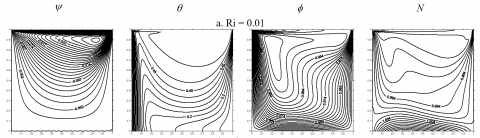## 3b.png## 3c.png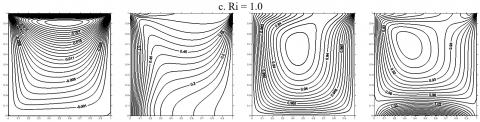## 3d.pngFigure 3. Streamlines, isotherms, iso-concentrations and isolines of micro- rotation for $\Phi ={{0}^{{}^\circ }},Ra={{10}^{4}},Rb=1,Sc=1,\chi =1,\Pr =6.2$, s1=1, Da=10-3

It can be observed that the values of the local Nusselt number decreases as the Richardson number increases. So, the maximum value of the local Nusselt number corresponds to the smallest value of the Richardson number [Ri=0.01] (i.e., in the forced convection-dominant ranges) and vice versa. The reason of this behavior is due to the greatest effect of the lid-driven wall for low Richardson numbers [i.e., Ri=0.01]. Therefore, the velocity of the left side lid-driven wall increases significantly and leads to increase the forced convection effect on the local Nusselt number results. From the other side, it is noticed that the values of the (NuY) reach their maximum at [Y=1] and begin to decrease as the values of (Y) decrease. This is again due to the strong effect of the lid-driven velocity at [Y=1] as mentioned above.

4.3 Effect of Richardson number on the local Sherwood number

Figure 5 displays the local Sherwood number profiles along the heated wall for various Richardson numbers [Ri=0.01 to 2] and [Φ=0o, Ra=104, Rb=1, Sc=1, s1=1, Da=10-3, Pr=6.2 and χ=1]. It can be noticed that the values of the local Sherwood number decrease as the Richardson number increases. So, the maximum value of the local Sherwood number is at [Ri=0.01] while, the minimum one is at [Ri=2.0]. From the other hand, (ShY) reaches its maximum values at [Y=1] and begin to drop gradually as the values of (Y) decrease. Moreover, it can be observed from Figure 4, that all values of the local Sherwood number reach to the same value at [Y=0].

4.4 Effect of the Richardson number on the velocity profiles

Figure 6 displays the profiles of the vertical velocity component (V) at the horizontal mid – section (Y=0.5) for various Richardson numbers [Ri=0.01 to 2] and [Φ=0o, Ra=104, Rb=1, Sc=1, Pr=6.2 and χ=1]. It can be seen from this figure that the vertical velocity component increases with the increase of the Richardson number. This behavior of (V) can be noticed at the left lid-driven sidewall [X=0], while a reverse one can be noticed at the right sidewall [i.e., X=1].

4.5 Effect of the Rayleigh number on streamlines, isotherms, iso-concentrations and isolines of micro-rotation

Streamlines (first column), isotherms (second column), iso-concentrations (third column) and iso-lines of micro-rotation (fourth column) are shown in Figure 7 when the Rayleigh number varies from [Ra=102 to 105] and [Φ=0o , Ha=10, Rb=10, Sc=1, Pr=6.2, Ri=0.05, s1=1, Da=10-3 and c=1]. The flow field is represented by a symmetrical vortex inside the square cavity.

The flow circulation inside it begins when the hot fluid rises adjacent to the hot bottom wall as a result of the buoyancy force, then moves along the adiabatic left sidewall until it reaches the cold top wall, after that it moves along the adiabatic right sidewall before it comes back again to the hot bottom wall. When the Rayleigh number is low (i.e., Ra=102 and 103), the re-circulating vortices inside the square cavity are symmetrical to each other due to the weak effect of the buoyancy force. Therefore, the behavior of streamlines and the flow field does not change significantly when the Rayleigh number is low. In this case, the natural convection contribution in the heat transfer mechanism is slight. As the Rayleigh number increases (i.e., Ra=104 and 105), the buoyancy force becomes stronger and the flow circulation inside the square cavity increases. Also, it can be noticed at [Ra=105] that the center of the re-circulating vortices begins to move in the right upper corner of the cavity and becomes more irregular in comparison with the corresponding vortices when the Rayleigh number is low. In this case, the buoyancy force is more dominant than viscous force.

For isotherms and iso-concentration contours, when the Rayleigh number is low (i.e., Ra=102 and 103), they are symmetrical and parallel to the cold top wall, indicating that most of the heat is transferred by the conduction and the double-diffusive heat transfer is poor. As the Rayleigh number increases (i.e., Ra=104 and 105 ), both the isotherms and iso-concentrations begin to spread out in the cavity and they change from the parallel shape to the irregular one indicating that the convection is the dominating heat transfer mechanism in the cavity and in this case the double-diffusive heat transfer is effective.

## 4.png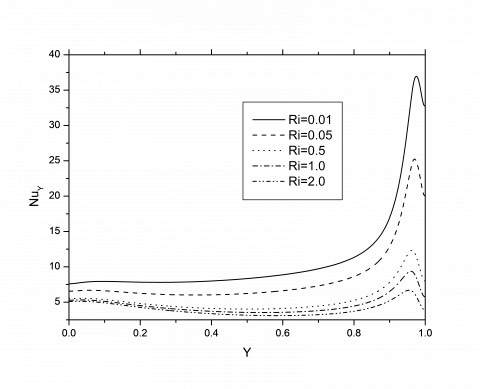Figure 4. Profiles of the local Nusselt number along the heated wall for various Richardson numbers at [Φ=0o, Ra=104, Rb=1, Sc=1, Pr=6.2, s1=1, Da=10-3 and χ=1]

## 5.png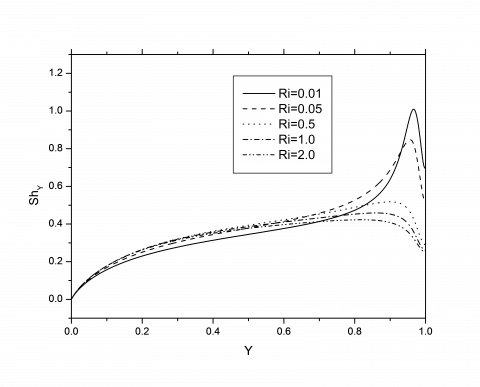Figure 5. Profiles of the local Sherwood number along the heated wall for various Richardson numbers at [Φ=0o, Ra=104, Rb=1, Sc=1, Pr=6.2, s1=1, Da=10-3 and χ=1]

## 6.png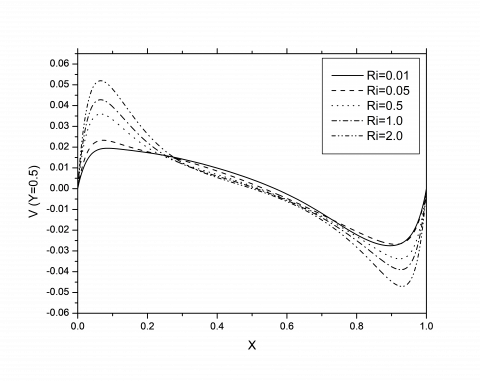Figure 6. Vertical  velocity component profiles at  the horizontal mid – section (Y=0.5) for various Richardson numbers and [Φ=0o, Ra=104, Rb=1, Sc=1, Pr=6.2, s1=1, Da=10-3 and χ=1]

Moreover, both the isotherms and iso-concentrations are crowded around the hot bottom wall. Now, in the next paragraph the effect of the Rayleigh number on the isolines of micro-rotation is discussed. It can be found from the results that the Rayleigh number has an important effect on these contours. When the Rayleigh number is low (i.e., Ra=102 and 103), the contours near the bottom wall are uniform, symmetrical and the magnitudes of the rotations begin to decrease as the Rayleigh number increases. From another side, the iso-lines of micro- rotation in the center of the cavity and near the top wall are greatly affected by the increase in the Rayleigh number from [Ra=102] to [Ra=103]. While, the values of the rotation begin to increase as the Rayleigh number increases. As the Rayleigh number increases (i.e., Ra=104 and 105), the isolines of micro-rotation refer that they begin to strongly clustered near the bottom wall and their values are still decreasing with the increase in the Rayleigh number. Also, a very clear confusion can be seen in the structure of isolines of micro- rotation in the cavity center and near the top wall and their values increase with the increase in the Rayleigh number. Therefore, it can be concluded that the isolines of micro- rotation are greatly influenced by the Rayleigh number especially in the center and the top wall of the cavity.

4.6 Effect of the Rayleigh number on the local Nusselt and Sherwood numbers

Figures. 8 and 9 display respectively the local Nusselt and Sherwood numbers profiles along the heated wall in the square cavity filled with a gyrotactic micro-organisms for various Rayleigh numbers [Ra=102 to 105] and [Φ=0o, Ha=10, Rb=10, Sc=1, Pr=6.2, Ri=0.05, s1=1, Da=10-3 and χ=1]. It can be seen that values of the local Nusselt and Sherwood numbers increase as the Rayleigh number increases. So, the maximum value of both of them corresponds to the greatest value of the Rayleigh number [Ra=105] (i.e., when the natural convection is dominant), while the minimum value of them corresponds to the smallest value of the Rayleigh number [Ra=102] (i.e., when the conduction is dominant). The reason of this behavior is due to the greatest effects of the thermal and solutal buoyancy forces when the Rayleigh number is high [i.e., Ra=105]. Again, the maximum values of (Nu Y) and (Sh Y) are noticed at [Y=1]. Also, the difference between the behaviors of both local Nusselt and Sherwood numbers is that in the local Nusselt number the profiles does not meet in the same point, while in the second one all the profiles are meet in the same point at [Y=0].

4.7 Effect of the Rayleigh number on the velocity profiles

Figure 10 explains the profiles of the vertical velocity component (V) at  the horizontal mid – section (Y=0.5) in the square cavity filled with a gyrotactic micro-organisms for various Rayleigh numbers [Ra=102 to 105] and [Φ=0o, Ha=10, Rb=10, Sc=1, Pr=6.2, Ri=0.05, s1=1, Da=10-3 and χ=1]. It can be seen from this figure that the vertical velocity component at [X=0] increases with the increase of the Rayleigh number. After that, it begins to decrease especially at [X=0.5] and finally reaches its zero value at [X=1].

4.8 Effects of the Richardson and Hartmann numbers on the average Nusselt and Sherwood numbers

The average Nusselt and Sherwood numbers profiles in the square cavity filled with a gyrotactic micro-organisms for various Richardson numbers [Ri=0.01 to 1 ] and Hartmann numbers [Ha=0 to 50 ] at  [Φ=0o, Ra=104, Rb=10, Sc=1, Pr=6.2, s1=1, Da=10-3 and χ=1] are explained in Figures 11 and 12 respectively.

It can be observed that the values of the average Nusselt number increase as the Richardson number decreases and decrease as the Hartmann number increases. This is due to the dominant effect of the lid-driven left sidewall when the Richardson number is very low [i.e., Ri=0.01] which leads to increase the share of the forced convection in the heat transfer process and leads subsequently to improve the average Nusselt number results. From the opposite side, when the Hartmann number increases the average Nusselt number decreases. This is due to the increase of the magnetic force effect when the Hartmann number increases. Also, the maximum value of the average Nusselt numbers occurs when the Hartmann number is zero (i.e., the magnetic field is absent). Therefore, the high average Nusselt numbers correspond to the low Hartmann number and vice versa. This is because the effect of the magnetic field becomes negligible when the Hartmann number is zero. Therefore, the flow circulation and the temperature gradient increase and for this reason the average Nusselt number increases. An opposite behavior can be found when the Hartmann number increases. With respect to the effect of both Richardson and Hartmann numbers on the average Sherwood number, it can be noticed from Figure 12 that the values increase as the Richardson number increases and exhibit different behaviors as the Hartmann number increases. For more explanations, they increase rapidly at [Ri=0.01] and slowly at [Ri=0.05 and 0.5] and exhibit a wavy behavior at [Ri=1].

4.9 Effects of the Rayleigh and Hartmann numbers on the average Nusselt and Sherwood numbers

The average Nusselt and Sherwood numbers profiles in the square cavity filled with nanofluid for various Rayleigh numbers [Ra=102 to 105] and Hartmann numbers [Ha=0 to 50] at [Φ=0o, Ri=0.05, Rb=10, Sc=1, Pr=6.2, s1=1, Da=10-3 and χ=1] are explained in Figures 13 and 14 respectively. It is clearly seen that the average Nusselt number values (Figure 13) increase as the Rayleigh number increases and decrease as the Hartmann number increases. From this figure, it is noticed that they increase strongly when the Rayleigh number increases, especially when the Hartmann number is zero (Ha=0). This is due to the increase in the convection currents intensity which causes a clear improvement in the average Nusselt number. However, for very low Rayleigh number (Ra=102), the average Nusselt number is almost invariant with the increase of (Ha). This is because the buoyancy force is weak, so the convection heat transfer is very slight and the conduction is dominated. It appears clearly from Figure 13 that the average Nusselt number decreases when Hartmann number increases. Since, the magnetic force is the dominant force and controls the flow inside the square cavity which leads to drop the average Nusselt number. With respect to the behavior of the average Sherwood number (Figure 14), it is clearly observed that it increases as the Rayleigh number increases from [Ra=102 to 104]. While, it decreases at [Ra=105]. This is because of high increasing in the solutal buoyancy force in this range of the Rayleigh number which causes a clear enhancement in the mass transfer inside the cavity and increases the average Sherwood number. But, at [Ra=105] a drop in the solutal buoyancy force occurs which leads to decrease the average Sherwood number at this value. Now to discuss the effect of the Hartmann number on the average Sherwood number profiles.

It is noticed that they decreased as the Hartmann number increases and this occurs for low range of the Rayleigh number [Ra=102 and 103]. An opposite behavior is seen for high Rayleigh number [Ra=104 and 105].

The average Nusselt and Sherwood numbers profiles in the square cavity filled with a gyrotactic micro-organisms for various Richardson numbers [Ri = 0.01 to 1] and magnetic field inclination angle [Φ= 0o to 350o] at [Ha=10, Ri=0.05, Ra=104, Sc=1, Pr=6.2, s1=1, Da=10-3 and χ=1] are shown in Figures 15 and 16 respectively. It can be seen that the average Nusselt number increases as the Richardson number decreases. No significant variation is observed when the magnetic field inclination angle increases. For the average Sherwood number, it increases as the Richardson number decreases. Again, no sensible variation was seen when the magnetic field inclination angle increases and the Richardson number varied from [Ri=0.05 to 1]. But a wavy variation was seen for very low Richardson number [i.e., Ri=0.01].

4.10 Effects of the bio-convection Rayleigh and Hartmann numbers on the average Nusselt and Sherwood numbers

The average Nusselt and Sherwood numbers profiles in the square cavity filled with a gyrotactic micro-organisms for various bio-convection Rayleigh numbers [Rb=10 to 40] and Hartmann numbers [Ha=0 to 50] at [Φ=0o, Ri=0.05, Ra=104, Sc=1, Pr=6.2, s1=1, Da=10-3 and χ=1] are explained in Figures 17 and 18 respectively.

## 7a.png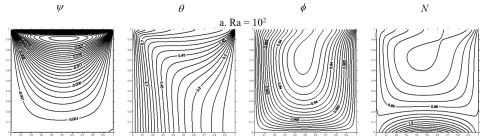## 7b.png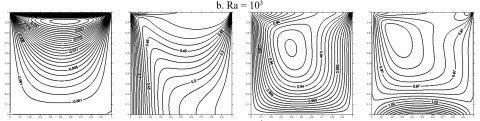## 7c.png## 7d.png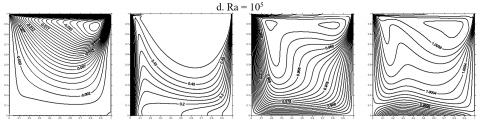Figure 7. Streamlines, isotherms , iso-concentrations  and isolines of micro- rotation for [Φ=0o, Ha=10, Rb=10, Sc=1, Pr=6.2, Ri=0.05, s1=1, Da=10-3 and χ=1]

## 8.png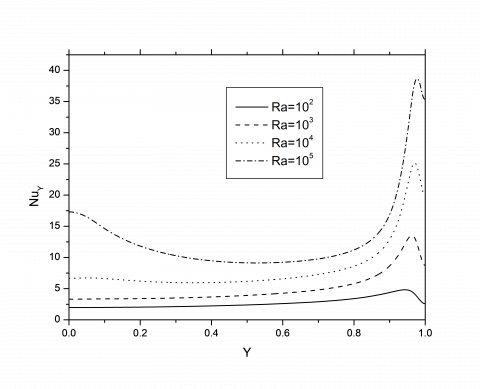Figure 8. Profiles of the local Nusselt number along the heated wall for various Rayleigh numbers at [Φ=0o, Ha=10, Rb=10, Sc=1, Pr=6.2, Ri=0.05, s1=1, Da=10-3 and χ=1]

## 9.png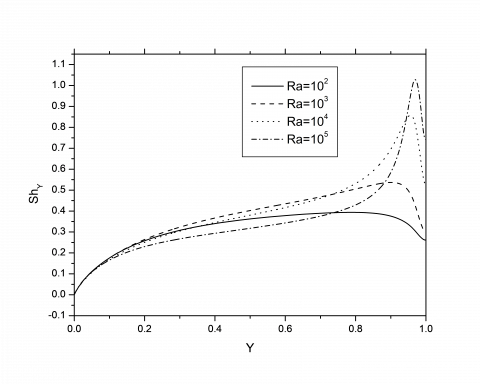Figure 9. Profiles of the local Sherwood number along the heated wall for various Rayleigh numbers at [Φ=0o, Ha=10, Rb=10, Sc=1, Pr=6.2, Ri=0.05, s1=1, Da=10-3 and χ=1]

## 10.png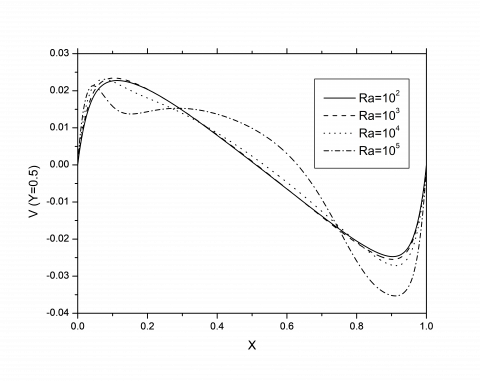Figure 10. Vertical velocity component profiles at the horizontal mid – section (Y=0.5) for various Rayleigh numbers and [Φ=0o , Ha=10, Rb=10, Sc=1, Pr=6.2, Ri=0.05, s1=1, Da=10-3 and χ=1]

## 11.png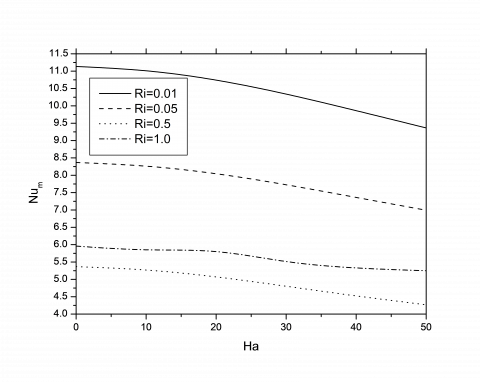Figure 11. Profiles of the average Nusselt number for various Richardson and Hartmann numbers at [Φ=0o, Ra=104, Rb=10, Sc=1, Pr=6.2, s1=1, Da=10-3 and χ=1]

## 12.png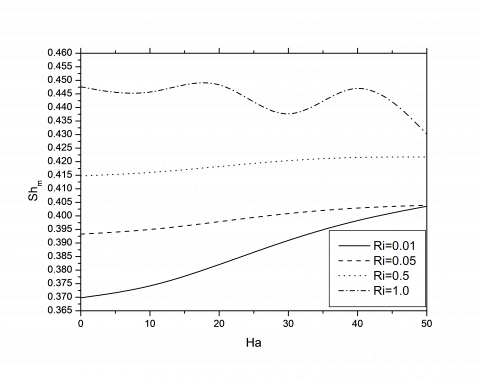Figure 12. Profiles of the average Sherwood number for various Richardson and Hartmann numbers at [Φ=0o, Ra=104, Rb=10, Sc=1, Pr=6.2, s1=1, Da=10-3 and χ=1]

## 13.png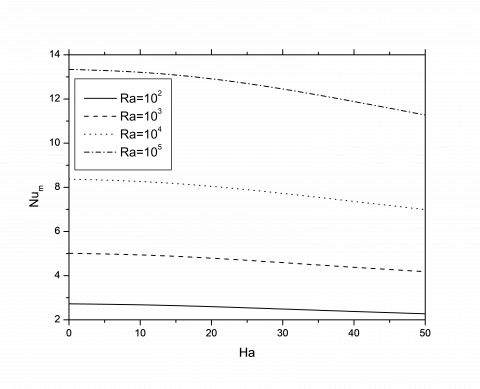Figure 13. Profiles of the average Nusselt number for various Rayleigh and Hartmann numbers at [Φ=0o, Ri=0.05, Rb=10, Sc=1, Pr=6.2, s1=1, Da=10-3 and χ=1]

## 14.png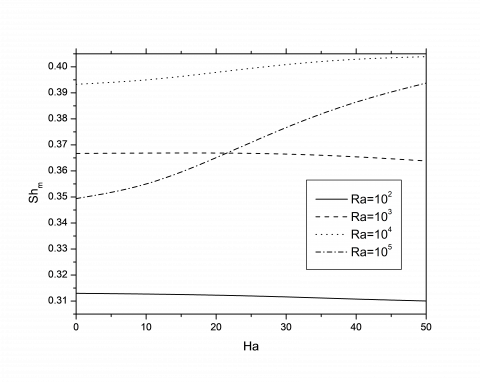Figure 14. Profiles of the average Sherwood number for various  Rayleigh and Hartmann numbers at [Φ=0o, Ri=0.05, Rb=10, Sc=1, Pr=6.2, s1=1, Da=10-3 and χ=1]

4.11 Effects of the Richardson number and the magnetic field inclination angle on the average Nusselt and Sherwood numbers

## 15.pngFigure 15. Profiles of the average Nusselt number for various Richardson number and magnetic field inclination angle at [Ha=10, Ri=0.05, Ra=104, Sc=1, Pr=6.2, s1=1, Da=10-3 and χ=1]

## 16.pngFigure 16. Profiles of the average Sherwood number for various Richardson number and magnetic field inclination angle at [Ha=10, Ri=0.05, Ra=104, Sc=1, Pr=6.2, s1=1, Da=10-3 and χ=1]

It is shown that the average Nusselt number values (Figure 17) increase as the bio-convection Rayleigh number decreases. Also, they decrease as the Hartmann number increases. The maximum value of the average Nusselt number can be noticed when the Hartmann number is zero. The lower heat transfer occurs for highest Hartmann number [Ha=50]. The reason of this behavior is due to the severe effect of the magnetic field for high Hartmann number which leads to drop the average Nusselt number values. From the opposite side, for (Ha=0) the magnetic field is absent and for this reason it leads to jump the average Nusselt number values. With respect to the results of the average Sherwood number (Figure 18), it is observed that it increases as the bio-convection Rayleigh number increases from [Rb=10 to 40]. Therefore, it can be concluded that the increase in (Rb) values leads to enhance the mass transfer in the cavity which is represented by the average Sherwood number. From another side, it can be seen that the average Sherwood number increases as the Hartmann number increases. This interesting result refers that the magnetic field has a positive effect on the average Sherwood number in contrast to the results seen of the average Nusselt number. However, some values of the average Nusselt and Sherwood numbers with the parameters which are discussed in the previous sections at [Sc=1, Pr=6.2 and χ=1] are illustrated in Table 2.

## 17.png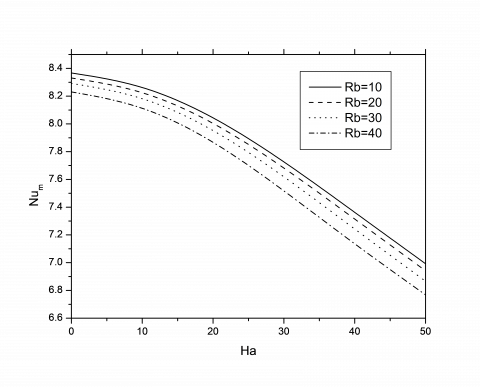Figure 17. Profiles of the average Nusselt number for various bio-convection Rayleigh and Hartmann numbers at  [Φ=0o, Ri=0.05, Ra=104, Sc=1, Pr=6.2, s1=1, Da=10-3 and χ=1]

## 18.png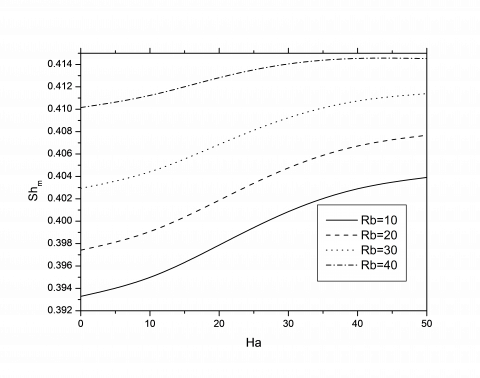Figure 18. Profiles of the average Sherwood number for various bio-convection Rayleigh and Hartmann numbers at [Φ=0o, Ri = 0.05, Ra=104 , Sc=1, Pr=6.2, s1=1, Da=10-3 and χ=1]

4.12 Effect of bio-convection Rayleigh number on the velocity profiles

Figure 19 displays the profiles of the vertical velocity component (V) at  the horizontal mid – section (Y=0.5) in the square cavity filled with nanofluid for various bio-convection Rayleigh numbers [Rb=10 to 40] and [Φ=0o, Ri=0.05, Ra=104, Ha=10, Sc=1, Pr=6.2, s1=1, Da=10-3 and χ=1]. It can be seen from this figure that the vertical velocity component at [X=0] increases with the reduction of the bio-convection Rayleigh number. While, a reverse behavior was seen at [X=0.5] until it reaches its zero value at [X=1].

Table 2. Values of the average Nusselt and Sherwood numbers for $Sc=1,\chi =1,\Pr =6.2$, s1=1, Da=10-3

 Ri Ra Rb Pe Ha Φ $N{{u}_{m}}$ $S{{h}_{m}}$ 0.01 104 ----   102 ---   104 10 ---- ------ ----- 100   10 0.1 ----   ----- -----   ----- 0 10 50 ----- -----   ---- π/2 ----- ----   -------     0 11.1366 11.1303 10.7739 3.8064 3.8063     9.3648 0.3697 0.3707 0.3976 0.3324 0.3408     0.4035 0.05 104 ---- ----- 10 ----- --- 0.1 0.1 ---- 0 10 50 π/2 ---- 0 8.3674 8.0183 6.9923 0.3932 0.3294 0.4039 0.5 104 ---- --- 10 ---- ---- 0.1 ----- ---- 50 100 50 π/2   0 5.3501 3.5047 4.2670 0.4150 0.4144 0.4217

## 19.png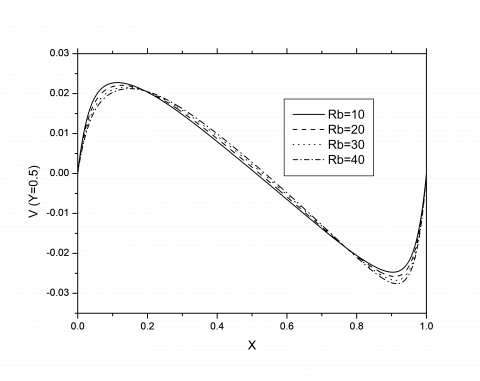Figure 19. Vertical velocity component profiles at the horizontal mid – section (Y=0.5) for various bio-convection Rayleigh numbers  and [Φ=0o, Ri=0.05, Ra=104, Ha=10, Sc=1, Pr=6.2, s1=1, Da=10-3 and χ=1]

5. Conclusion

The main results of this study can be summarized as:

- For [Ri=0.01 and 0.5], the absolute values of the stream function begin to decrease as the Richardson number increases and the convection heat transfer is dominant. Also, iso-concentrations adjacent the hot bottom wall, are greater than their values at the cold top one. Moreover, as the Richardson number increases, the strength of the microrotation decreases in the core of the cavity. A reverse behavior is noticed adjacent the hot bottom wall.

- No significant variations can be seen on the flow, thermal and solutal fields at [Ri = 1].

- For [Ri=2.0], the natural convection effect increases and the transferred heat and mass energies by them increase also which leads to increase the rate of heat and mass transfer inside the cavity. Also, the strength of the microrotation decreases in the core of the cavity. While, it increases adjacent the hot bottom wall.

-The local Nusselt and Sherwood numbers along the heated wall decrease as the Richardson number increases and increase as the Rayleigh number increases.

-Vertical velocity component at (Y=0.5) increases with the increase of (Ri) at the left lid-driven sidewall [X=0], while a reverse one can be noticed at the right one [X=1].

-Vertical velocity component at (Y=0.5) increases with the increase of (Ra) at [X=0]. After that, it begins to decrease especially at [X=0.5] and finally reaches its zero value at [X=1].

-The average Nusselt number increases as the Richardson number decreases.

-The average Sherwood number increases as the Richardson number increases. While, they exhibit different behaviors as the Hartmann number increases.

-The average Nusselt number increases with Rayleigh number and decrease with Hartmann increase. For the average Sherwood number, it increases as the Rayleigh number increases from [Ra=102 to 104] but, it decreases at [Ra=105]. While, it decreases as the Hartmann number increases for [Ra=102 and 103] and increases for [Ra=104 and 105].

-No significant variation was seen in the average Nusselt number when the magnetic field inclination angle increases. Also, no sensible variation in the average Sherwood number was seen when this angle increases and [Ri=0.05 to 1]. But a wavy variation was observed at [Ri=0.01].

-When the bio-convection Rayleigh number increases the average Nusselt number decreases while the average Sherwood number increases.

-Vertical velocity component at (Y=0.5) increases with decrease of (Rb) at [X=0]. While, a reverse behavior was seen at [X=0.5] until it reaches its zero value at [X=1].

-When the Rayleigh number increases, the flow circulation and the convection effect increase significantly. Also, the double-diffusive heat transfer becomes more effective.

- Isolines of micro-rotation are greatly influenced by the Rayleigh number especially in the center and the top wall of the cavity.

References

 Iwatsu, R., Hyun, J., Kuwahara, K. (1993). Mixed convection in a driven cavity with a stable vertical temperature gradient. International Journal of Heat and Mass Transfer, 36(6): 1601-1608. https://doi.org/10.1016/S0017-9310(05)80069-9

 Ouertatani, N., Ben Cheikh, N., Ben Beyaa, B., Lilia, T., Campo, A. (2009). Mixed convection in a double lid-driven cubic cavity. International Journal of Thermal Sciences, 48(7): 1265-1272. https://doi.org/10.1016/j.ijthermalsci.2008.11.020

 Khanafer, K., Chamkha, A.J. (1999). Mixed convection flow in a lid-driven enclosure filled with a fluid-saturated porous medium. International Journal of Heat and Mass Transfer, 42(13): 2465-2481. https://doi.org/10.1016/S0017-9310(98)00227-0

 Al-Rashed, A.A.A.A., Kalidasan, K., Kolsi, L., Velkennedy, R., Aydi, A., Hussein, A.K., Malekshah, E.H. (2018). Mixed convection and entropy generation in a nanofluid filled cubical open cavity with a central isothermal block. International Journal of Mechanical Sciences, 135: 362-375. https://doi.org/10.1016/j.ijmecsci.2017.11.033

 Kolsi, L., Oztop, H.F., Abu-Hamdeh, N., Borjini, M.N., Ben Aissia, H. (2017). Effects of moving lid direction on mixed convection and entropy generation in a cubical cavity with longitudinal triangular fin insertion. International Journal of Numerical Methods for Heat & Fluid Flow, 27(4): 839-860. https://doi.org/10.1108/HFF-02-2016-0054

 Kolsi, L. (2016). MHD mixed convection and entropy generation in a 3D lid-driven cavity. Frontiers in Heat and Mass Transfer, 7(26): 1-10. https://doi.org/10.1108/10.5098/hmt.7.26

 Kolsi, L., Oztop, H.F., Borjini, M.N., Al-Salem, K. (2011). Second law analysis in a three dimensional lid-driven cavity. International Communications in Heat and Mass Transfer, 38(10): 1376-1383. https://doi.org/10.1016/j.icheatmasstransfer.2011.08.019

 Saha, S., Saha, G., Ali, M., Islam, M.Q. (2007). Combined free and forced convection inside a two-dimensional multiple ventilated rectangular enclosure. ARPN Journal of Engineering and Applied Sciences, 1: 23-35.

 Rahman, M.D.M., Alim, M.A., Saha, S., Chowdhury, M.K. (2008). A numerical study of mixed convection in a square cavity with a heat conducting square cylinder at different locations. Journal of Mechanical Engineering, 39(2): 78-85. http://dx.doi.org/10.3329/jme.v39i2.1850

 Nield, D.A., Bejan, A. (2013). Convection in Porous Media. Springer, 4th edition.

 Vafai, K. (2005). Handbook of Porous Media. 2nd ed. Taylor & Francis, New York.

 Pop, I., Ingham, D.B. (2001). Convective heat transfer: Mathematical and computational modelling of viscous fluids and porous media. Pergamon, Oxford.

 Ingham, D.B., Pop, I. (1998). Transport Phenomena in Porous Media. Pergamon, Oxford.

 Pedley, T.J., Kessler, J.O. (1992). Hydrodynamic phenomena in suspensions of swimming microorganisms. Annual Review of Fluid Mechanics, 24: 313-358. https://doi.org/10.1146/annurev.fl.24.010192.001525

 Hillesdon, A.J., Pedley, T.J. (1996). Bioconvection in suspensions of oxytactic bacteria: linear theory. Journal of Fluid Mechanics, 324: 223-259. https://doi.org/10.1017/S0022112096007902

 Kuznetsov, A.V. (2006). The onset of thermos-bioconvection in a shallow fluid saturated porous layer heated from below in a suspension of oxytactic microorganisms. European Journal of Mechanics - B/Fluids, 25(2): 223-233. https://doi.org/10.1016/j.euromechflu.2005.06.003

 Bég, O.A., Zueco, J., Norouzi, M., Davoodi, M., Joneidi, A.A., Elsayed, A.F. (2014). Network and Nakamura tridiagonal computational simulation of electrically-conducting biopolymer micro-morphic transport phenomena. Computers in Biology and Medicine, 44: 44-56. https://doi.org/10.1016/j.compbiomed.2013.10.026

 Alloui, Z., Nguyen, T.H., Bilgen, E. (2007). Numerical investigation of thermo-bioconvection in a suspension of gravitactic microorganisms. International Journal of Heat and Mass Transfer, 50(7-8): 1435–1441. https://doi.org/10.1016/j.ijheatmasstransfer.2006.09.008

 Alloui, Z., Nguyen, T.H., Bilgen, E. (2006). Stability analysis of thermobioconvection in suspensions of gravitactic microorganisms in a fluid layer. International Communications in Heat and Mass Transfer, 33(10): 1198-1206. https://doi.org/10.1016/j.icheatmasstransfer.2006.08.012

 Kuznetsov, A.V. (2011). Bio-thermal convection induced by two different species of microorganisms. International Communications in Heat and Mass Transfer, 38(5): 548-553. https://doi.org/10.1016/j.icheatmasstransfer.2011.02.006

 Kuznetsov, A.V., Avramenko, A.A., Geng, P. (2004). Analytical investigation of a falling plume caused by bioconvection of oxytactic bacteria in a fluid saturated porous medium. International Journal of Engineering Science, 42(5-6): 557-569. https://doi.org/10.1016/j.ijengsci.2003.08.004

 Kuznetsov, A.V. (2006). The onset of thermo-bioconvection in a shallow fluid saturated porous layer heated from below in a suspension of oxytactic microorganisms. European Journal of Mechanics B/Fluids, 25(2): 223–233. https://doi.org/10.1016/j.euromechflu.2005.06.003

 Kuznetsov, A.V. (2005). The onset of bioconvection in a suspension of gyrotactic microorganisms in a fluid layer of finite depth heated from below. International Communications in Heat and Mass Transfer, 32: 574-582. https://doi.org/10.1016/j.icheatmasstransfer.2004.10.021

 Kuznetsov, A.V. (2005). Thermo-bioconvection in a suspension of oxytactic bacteria. International Communications in Heat and Mass Transfer, 32: 991-999. https://doi.org/10.1016/j.icheatmasstransfer.2004.11.005

 Chamkha, A.J., Rashad, A.M., Kameswaran, P.K., Abdou, M.M.M. (2017). Radiation effects on natural bioconvection flow of a nanofluid containing gyrotactic microorganisms past a vertical plate with streamwise temperature variation. Journal of Nanofluids, 6(3): 587-595. https://doi.org/10.1166/jon.2017.1351

 Al-Mudhaf, A.F., Chamkha, A.J., Rashad, A.M., Abdou, M.M.M. (2019). Mixed bioconvection flow over a wedge in porous media saturated with a nanofluid containing both nanoparticles and gyrotactic microorganisms. Journal of Porous Media, (In Press). https://doi.org/10.1016/j.compbiomed.2013.10.026

 Patankar, S.V. (1980). Numerical Heat Transfer and Fluid Flow. Hemisphere Publishing Corporation, Taylor and Francis Group, New York.